# Jun 7, Worksheet on Word Problems on Addition and Subtraction Together | Ans

## Jun 7, Worksheet on Word Problems on Addition and Subtraction Together | Ans

Table of Contents

In 4th grade worksheet on word problems on addition and subtraction, all grade students can practice the questions on word problems based on addition and subtraction. This exercise sheet on addition and subtraction can be practiced by the students to get more ideas to solve the worksheet on word problems on addition and subtraction.

1. What is the sum of 40711 and 73412 and the difference of 82731 and 37128?

2. Find the number which is:

(i) 53172 more than 64278

(ii) 53172 less than 64278

(iii) 1872 more than 23265

(iv) 1872 less than 23265

3. Find the number which exceeds

(i) 17319 by 1279 and

(ii) 23735 by 2035.

4. (i) Which number should be added to 25483 to get the sum of 65785?

(ii) Which number should be subtracted from 52847 to get 22489?

5. How much smaller is 63512 than 94291?

6. Which is greater, 42929 or 91429 and by how much?

7. (i) Find the difference of the two sums, 14581 + 18541 and 23427 + 23243.

(ii) Find the sum of the two differences, 32309 – 23903 and 41724 – 31527.

8. A milk-dairy produces 25,545 litres of milk every day. It supplies 15,625 litres of milk to a milk-depot and the rest to the market. How much milk is supplied to the market?

9. The sum of two numbers is 94506. One of the numbers is 49605. Find the other number.

10. The sum of two numbers is 45000. One of the numbers is 22500. Find the other number. Which part of the sum is the given number?17. Team A scored 478 runs in first innings and 345 runs in
second innings. Team B scored 389 runs in first innings and 472 runs in second
innings. Which team won the match and by how many runs?

Answer: Team B, 38 runs

18. Jenny reads 324 pages from her book on Saturday and 259
pages on Sunday. There are 876 pages in the book. How many more pages she has
to read?

Answer: 293

If students have any queries regarding the questions in the worksheet on word problems on addition and subtraction please fill-up the below comment box so that we can help you. However, suggestions for further improvement, from all quarters would be greatly appreciated.

## You might like these

•### Estimating Sums and Differences | Estimations | Practical Calculations

For estimating sums and differences in the number we use the rounded numbers for estimations to its nearest tens, hundred, and thousand. In many practical calculations, only an approximation is required rather than an exact answer. To do this, numbers are rounded off to a

•### Worksheet on Forming Numbers with Digits | Smallest & Greatest Numbers

In the worksheet on forming numbers with digits, the questions will help us to practice how to form different types of smallest and greatest numbers using different digits. We know that all the numbers are formed with the digits 0, 1, 2, 3, 4, 5, 6, 7, 8 and 9.

•### Worksheets on Comparison of Numbers | Find the Greatest Number

In worksheets on comparison of numbers students can practice the questions for fourth grade to compare numbers. This worksheet contains questions on numbers like to find the greatest number, arranging the numbers etc…. Find the greatest number:

•### Formation of Greatest and Smallest Numbers | Arranging the Numbers

the greatest number is formed by arranging the given digits in descending order and the smallest number by arranging them in ascending order. The position of the digit at the extreme left of a number increases its place value. So the greatest digit should be placed at the

•### Even and Odd Numbers | Definition | Properties | Examples | Questions

A number which is a multiple of 2 is an even number and that which is not multiple of 2 is an odd number. All those numbers that can be put into pairs are called even numbers, that is, all those numbers which come in the table of two are even numbers.

•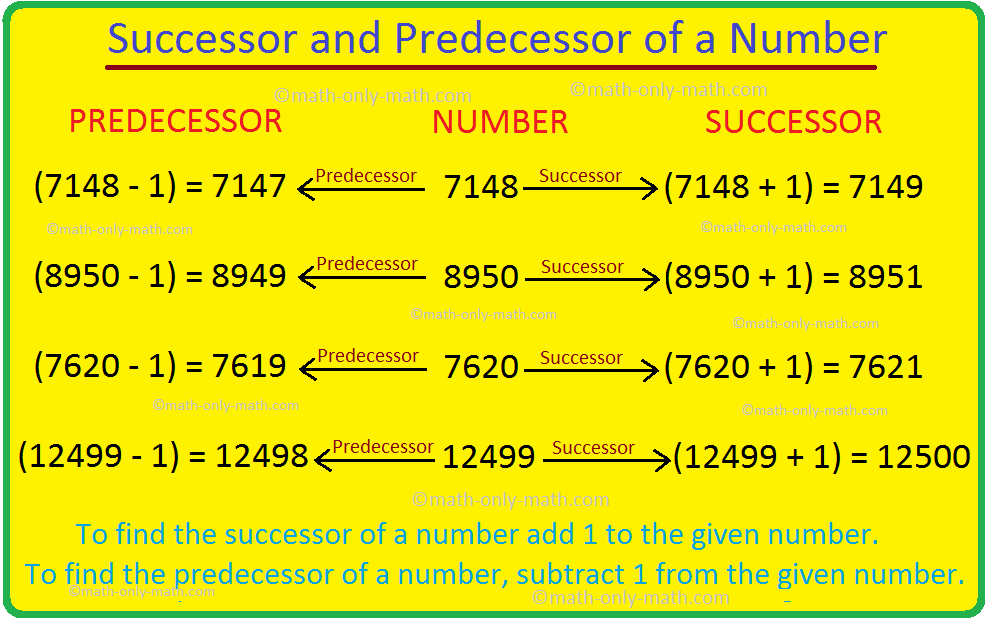### Successor and Predecessor | Successor of a Whole Number | Predecessor

The number that comes just before a number is called the predecessor. So, the predecessor of a given number is 1 less than the given number. Successor of a given number is 1 more than the given number. For example, 9,99,99,999 is predecessor of 10,00,00,000 or we can also

•### Worksheets Showing Numbers on Spike Abacus | Number in Figures

Worksheets showing numbers on spike abacus for 4th grade math questions to practice after learning 1 digit, 2 digits, 3 digits, 4 digits and 5 digits numbers on spike abacus.

•### Numbers Showing on Spike Abacus | Spike Abacus | Name of a Number

Numbers showing on spike abacus helps the students to understand the number and its place value. Spike abacus is very helpful to understand the concept of magnitude and name of a number.

•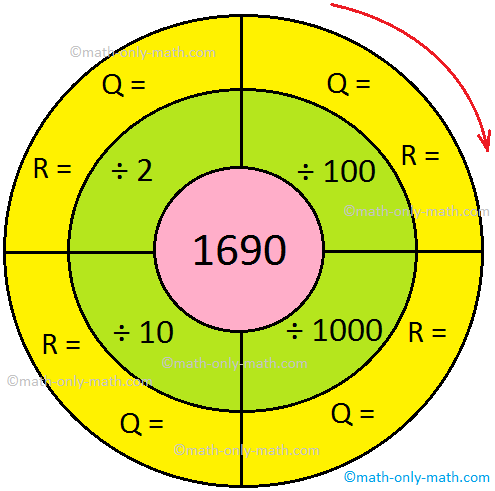### 4th Grade Division Worksheet | Simple Division | Math Division|Answers

In 4th grade division worksheet we will solve division by 2-digit numbers, division by 10 and 100, properties of division, estimation in division and word problems on division.

•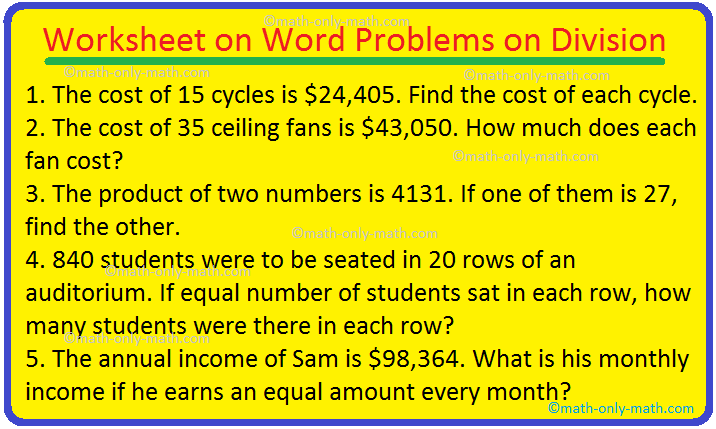### Worksheet on Word Problems on Division | Solve Division Problems

In worksheet on word problems on division, all grade students can practice the questions on word problems involving division. This exercise sheet on word problems on division can be practiced by the students to get more ideas to solve division problems.

•### Worksheet on Estimating the Quotient | Questions on Estimate Quotient

In worksheet on estimating the quotient, all grade students can practice the questions on estimate the quotient. This exercise sheet on estimating quotient can be practiced by the students to get more ideas. Find the estimated quotient for the following divisions:

•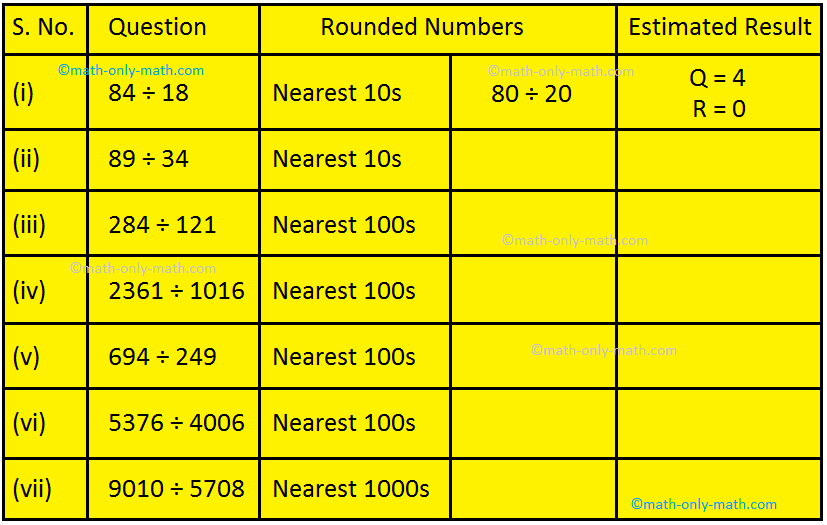### Estimating the Quotient | Process of Division | Estimation in Division

To estimate the quotient, we first round off the divisor and the dividend to the nearest tens, hundreds, or thousands and then divide the rounded numbers. In a division sum, when the divisor is made up of 2 digits or more than 2 digits, it helps if we first estimate the

•### Worksheet on Divide by 10, 100 and 1000 Divisors |Quotient & Remainder

Practice the questions given in the worksheet on divide by 10, 100 and 1000 divisors to find the quotient and remainder if any. Find the quotient and remainder (if any): I. Divide the given numbers by 10 and find the Quotient and Remainder. II. Divide the given numbers by

•### Worksheet on Division by Two-Digit Numbers | Find Quotient & Remainder

In worksheet on division by two-digit numbers, all grade students can practice the questions on dividing numbers by two digits numbers. This exercise sheet on dividing numbers can

•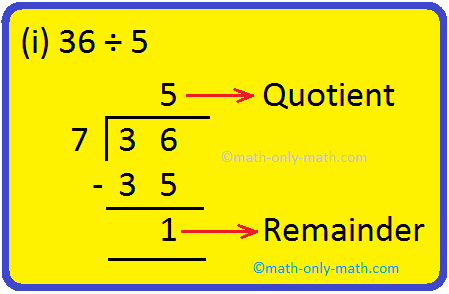### Worksheet on Division | Find out the Quotient and Remainder | Verify

In worksheet on division, all grade students can practice the questions to divide the numbers and find out the quotient and remainder. This exercise sheet on division can be practiced by the students to get more ideas to learn to divide and verify the results.

Four Fundamental Operations – worksheets

Worksheet on Addition.

Worksheet on Word Problems on Addition.

Worksheet on Subtraction.

Worksheet on Mixed Addition and Subtraction.

Worksheet on Word Problems on Addition and Subtraction.

Worksheet by Adding or Subtracting.

Worksheet on Addition and Subtraction.

Worksheet on Estimating Sums and Differences.

Worksheet on Multiplication.

Worksheet on Multiplication of a Number by a 2-Digit Number.

Worksheet on Multiplication of a Number by a 3-Digit Number.

Worksheet on Estimating Products.

Worksheet on Word Problems on Multiplication.

Worksheet on Division.

Worksheet on Division Facts.

Worksheet on Estimating the Quotient.

Worksheet on Dividing Numbers.

Worksheet on Division by Two-Digit Numbers.

Worksheet on Word Problems on Division.

Worksheet on Four Fundamental Operations.

Worksheet on Systems of Numeration.

4th Grade Math Activities

4th Grade Math Worksheets

From Worksheet on Word Problems on Addition and Subtraction to HOME PAGE

### New! Comments

Have your say about what you just read! Leave me a comment in the box below. Ask a Question or Answer a Question.

Didn’t find what you were looking for? Or want to know more information
about
Math Only Math.
Use this Google Search to find what you need.##### Also read : Interview QuestionsJun 7, Worksheet on Word Problems on Addition and Subtraction Together | Ans
#Jun #Worksheet #Word #Problems #Addition #Subtraction #Ans